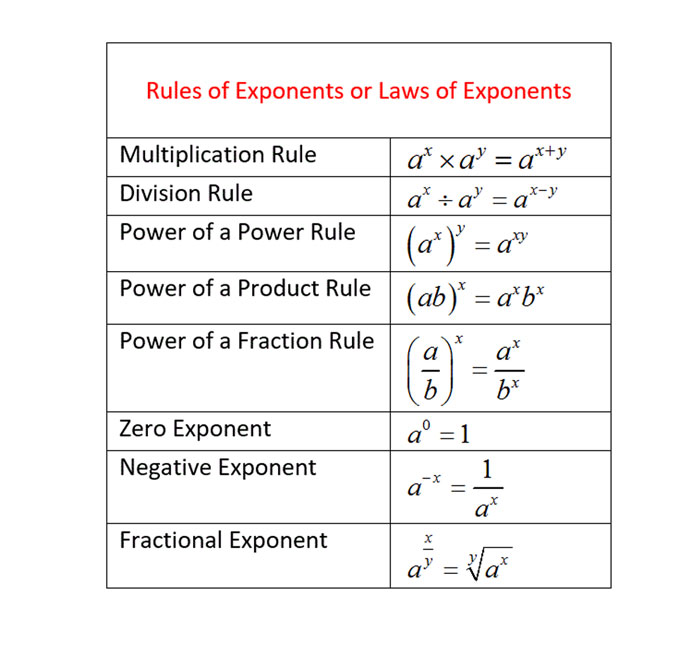# Rules of Exponents

We require a common language if we want to communicate mathematical concepts and ideas efficiently and clearly.

One example is Exponential Notation.

Question 1 of 3

1. Use rules of exponents and rewrite this expression as a single exponent.A.
B.
C.

Question 1 of 3

Question 2 of 3

2. Use rules of exponents and rewrite this expression as a single exponent.A.
B.
C.

Question 2 of 3

Question 3 of 3

3. Use rules of exponents and rewrite this expression as a single exponent.A.
B.
C.

Question 3 of 3

Next lesson: Scientific Notation

Continue learning.

This video transcript is for your convenience. This was developed to clearly express repeated multiplications and to simplify long numbers. As an example, often exponents are used in growth models for demographics and populations to manipulate and manage large numbers that are changing quickly over time.

So when we want to work with exponents efficiently, we should “speak that language” and get known with some rules first.

So what exactly is Exponential Notation?

Well, exponential notation consists of two parts. First, we have the base. As the name already suggests, this is the number found on the bottom. The second part of the exponential notation is the small number in superscript placed to the right of our base. It’s called the exponent. Listed here are a few examples of exponential notation. Let’s take a look at these examples to learn more about “exponential notation”:

 103 251 -34

Let’s begin with 103.  Our base is 10, meaning 10 (ten is a factor.  It will be multiplied by itself a certain number of times. This number of times is indicated by the exponent, which is the number written in superscript. Here, our exponent is three (3), meaning our base of 10 (ten) will be used 3 (three) times. So 103 is meaning: 10 • 10 • 10.

So now we understand what 103 is meaning, but how are we pronouncing this? We have quite a few choices. The term could be pronounced as “ten (10) raised to the 3rd power” or “ten (10) to the 3rd,” or “ten (10) cubed.”  The phrase “raised to a power” is inserted between our base and our exponent for the indication of exponential notation.

Okay so far. Now, let’s look at 251. What is an exponent of one (1) meaning? Well, any value that’s raised to the power of one (1) is only that value. This is making sense when thinking about it, as an exponent of one (1) is meaning that our base is used as a factor just once. So our base is standing alone, so 251 is just 25.

Now to the term -34. Well, this example may be a bit trickier because of the negative sign. One of the exponential notation rules is that exponents relate only to the values immediately to the left. So, -34 is not meaning -3 • -3 • -3 • -3. It is meaning: “the opposite of 34. Or — (3 • 3 • 3 • 3). Now if we would have wanted our base to be -3, we would have used parentheses in our notation: (-3)4.  Now, why are we so picky? Well, just do the math:

-34 equals – (3 • 3 • 3 • 3) = -81

(-3)equals -3 • -3 • -3 • -3 = 81

You see, that’s a huge difference.

Rules for Computing with Exponents

We’ve just learned that exponents only relate to numbers directly to their left unless we use parentheses. Here’s one more rule: when exponents are present outside parentheses, everything inside must be raised to that power. Let’s look at an example:

(5 + 3)2

In line with our order of operations, we first need to simplify stuff inside the parentheses before doing any other operation. So we’re adding 5 and 3 after which we can square that sum, eight (8), to get to our answer of 64. One more way to proceed is the following. Rewrite (5 + 3)as (5 + 3) times (5 + 3). Then, multiply this out to again reach 64.

(5 + 3)2 equals (8)2 which equals 8 • 8 which equals 64

(5 + 3)2 equals (5 + 3) times (5 + 3) which equals 5(5 + 3) + 3(5 +3) which equals 25 + 15 + 15 + 9 which is 64.

Parentheses may also be used in more ways with exponential notation. To give you an example, we may use parentheses to describe exponential terms to a power. Let’s look at 5and raise this to the fourth (4th) power. We would write this as (52)4. When numbers written in exponential notation are raised to a power, we call this a “power of a power.”

In the expression above, our base is 5and our exponent is 4. 5is used as a factor four (4) times. We may rewrite this problem also as: 52  52 • 52 •52, or (5 • 5) • (5 • 5) • (5 • 5) • (5 • 5).

Do you see that this works out to five (5) multiplied eight (8) times? Another way to write this is 58.

This brings us to one more rule. Compare this 58 to our original term: (52)4. Do you see that our new exponent is identical to our original exponents? Well, 2 • 4 = 8.

So we can simplify the power to a power by multiplying the exponents while keeping our base the same. We also have this rule if we want to combine two numbers in exponential notation that have identical bases. Look at the following expression:

(23)(24)

We can rewrite this as (2 • 2 • 2) times (2 • 2 • 2 • 2), or:  2 • 2 • 2 • 2 • 2 • 2 • 2. In exponential notation, we would write this product as 27. You may notice that seven (7) is the sum of our original two exponents three (3) and four (4). So if we want to multiply exponential terms that have identical bases, just add the exponents.

Rules of Exponents

Exponents apply only to the values to the immediate left.

When quantities in parentheses are raised to a power, exponents apply to everything inside those parentheses.

If we want to multiply two terms that have identical bases, add the exponents. (nx)(ny) equals nx+y

If we want to raise a power to a power, simply multiply both exponents. (nx)equals nxy